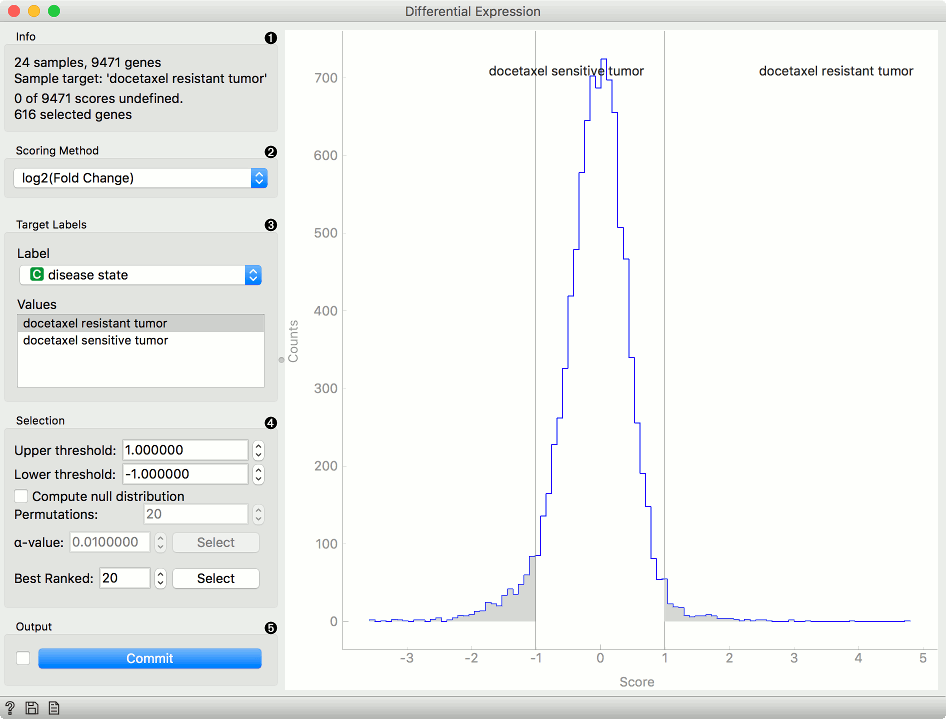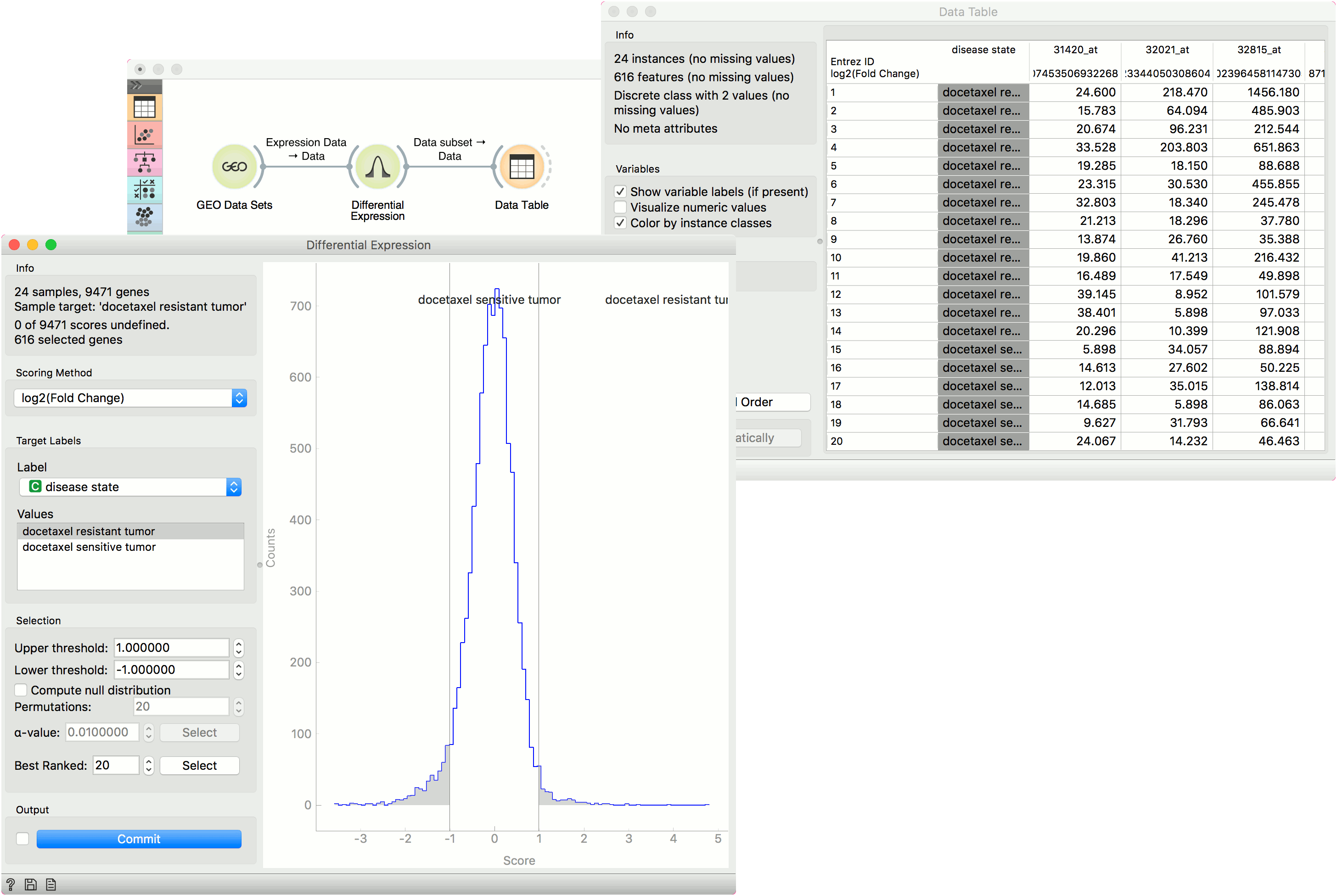# Differential Expression¶

Plots differential gene expression for selected experiments.

Inputs

• Data: Data set.

Outputs

• Data Subset: Differentially expressed genes.
• Remaining Data Subset: Genes that were not differentially expressed.
• Selected Genes: Genes from the select data with scores appended.

This widget plots a differential gene expression graph for a sample target. It takes gene expression data as an input (from dictyExpress, GEO Data Sets, etc.) and outputs a selected data subset (normally the most interesting genes).1. Information of the data input and output. The first line shows the number of samples and genes in the data set. The second line displays the selected sample target (read around which the graph is plotted). The third line shows the number of undefined genes (missing data) and the fourth the number of genes in the output.
2. Select the plotting method in Scoring method:
• Fold change: final to initial value ratio
• log2 (fold change): binary logarithmic transformation of fold change values
• T-test: parametric test of null hypothesis
• T-test (P-value): parametric test of null hypothesis with P-value as criterium
• ANOVA: variance distribution
• ANOVA (P-value): variance distribution with P-value as criterium
• Signal to NoiseRatio: biological signal to noise ratio
• Mann-Whitney: non-parametric test of null hypothesis with P-value as criterium
• Hypergeometric test: for binary expression data.
3. Select Target Labels. Labels depend on the attributes in the input. In Values you can change the sample target (default value is the first value on the list, alphabetically or numerically).
4. Selection box controls the output data.
• By setting the Lower threshold and Upper threshold values you are outputting the data outside this interval (the most interesting expression levels). You can also manually place the threshold lines by dragging left or right in the plot.
• If you click Compute null distribution box, the widget will calculate null distribution and display it in the plot. Permutations field allows you to set the precision of null distribution (the more permutations the more precise the distribution), while alpha-value will be the allowed probability of false positives. Press Select to output this data.
• The final option is to set the number of best ranked genes and output them with Select.
5. When Auto commit is on is ticked, the widget will automatically apply the changes. Alternatively press Commit. If the Add gene scores to output is ticked, the widget will append an additional column with gene scores to the data.

## Example¶

From the GEO Data Sets widget, we selected Breast cancer and docetaxel treatment (GDS360) with 14 treatment resistant and 10 treatment sensitive tumors. Then we used the Differential Expression widget to select the most interesting genes. We left the upper and lower threshold at default (1 and -1) and output the data. Then we observed the selected data subset in a Data Table. The table shows selected genes with an additional gene score label.